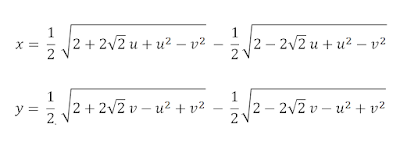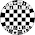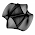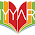## Thursday, September 3, 2015

### Mapping a Circle to a Square

Philip Nowell (http://mathproofs.blogspot.com/2005/07/mapping-square-to-circle.html)
devised equations for mapping a square to a circle. I will provide the inverse to the mapping below.

For this blog entry, I use a slightly different notation from his equations; i.e.
u = x'
v = y'

(u,v) are circular coordinates in the domain {(u,v) | u² + v² ≤ 1}
(x,y) are square coordinates in the range [-1,1] x [-1,1]

Here are the inverse mapping equations:

x = ½ √( 2 + 2u√2 + u² - v² ) - ½ √( 2 - 2u√2 + u² - v² )
y = ½ √( 2 + 2v√2 - u² + v² ) - ½ √( 2 - 2v√2 - u² + v² )

or more legiblyThe forward equations for the mapping are:

u = x √( 1 - ½ y² )
v = y √( 1 - ½ x² )

Here are some examples of the forward & inverse mappings at work.For a proof/derivation of the inverse equations, see my paper in
arxiv: "Analytical Methods for Squaring the Disc"

In this blog, I shall refer to this mapping as the elliptical grid mapping.  I chose this name because the mapping has a predilection to map a rectilinear grid into a grid of elliptical arcs in the circle.  See Nowell's blog for a discussion and derivation of the elliptical arcs.

Here are more examples of the mapping:Starbucks™ logo converted to a squareAndy Warhol's pop art "Marilyn" as a circle

[ C++ implementation source code below]
------------------------------------------------------------------------------------------

``````// sample code accompanying the paper:
// "Analytical Methods for Squaring the Disc"
// http://arxiv.org/abs/1509.06344``````
``````
``````
``````#include <stdio.h>
#include <math.h>
// Elliptical Grid mapping
// mapping a circular disc to a square region
// input: (u,v) coordinates in the circle
// output: (x,y) coordinates in the square
void ellipticalDiscToSquare(double u, double v, double& x, double& y)
{
double u2 = u * u;
double v2 = v * v;
double twosqrt2 = 2.0 * sqrt(2.0);
double subtermx = 2.0 + u2 - v2;
double subtermy = 2.0 - u2 + v2;
double termx1 = subtermx + u * twosqrt2;
double termx2 = subtermx - u * twosqrt2;
double termy1 = subtermy + v * twosqrt2;
double termy2 = subtermy - v * twosqrt2;
x = 0.5 * sqrt(termx1) - 0.5 * sqrt(termx2);
y = 0.5 * sqrt(termy1) - 0.5 * sqrt(termy2);

}

// Elliptical Grid mapping
// mapping a square region to a circular disc
// input: (x,y) coordinates in the square
// output: (u,v) coordinates in the circle
void ellipticalSquareToDisc(double x, double y, double& u, double& v)
{
u = x * sqrt(1.0 - y*y/2.0);
v = y * sqrt(1.0 - x*x/2.0);
}

int main()
{
double x,y;
double u,v;

ellipticalSquareToDisc(-0.789,0.654,u,v);
ellipticalDiscToSquare(u,v,x,y);

printf("%f %f\n",u,v);
printf("%f %f\n",x,y);

ellipticalDiscToSquare(0.31415, -0.926535,x,y);
ellipticalSquareToDisc(x,y,u,v);

printf("%f %f\n",x,y);
printf("%f %f\n",u,v);

return 0;
}

```
```

1.If u2 == v2, I find that termx2 and termy2 can be negative and result in errors.

1.I'm assuming you mean when u = v = sqrt(2) on the circle. The error you are getting with regards to a negative argument to a square root is because of numerical imprecision.  If you observe the value of termx2 and termy2 in the source code for the case u=v=sqrt(2), they are very small and close to zero.  You can insert this code before the square root calls in order to avoid numerical imprecision of floating point numbers.

double epsilon = 0.0000001;
if (fabs(termx2)< epsilon) {
termx2 = 0.0;
}
if (fabs(termy2)< epsilon) {
termy2 = 0.0;
}

In a perfect world of floating point numbers without numerical imprecision, termx2 and termy2 should be equal to zero for this case

2.This comment has been removed by the author.

3.thanks for the write up.
Made a javascript/Canvas2D based version on codepen
https://codepen.io/mxfh/pen/gRLqXX#https%3A%2F%2F2.bp.blogspot.com%2F-GaCwOyqLmjw%2FVfN3yDNgGCI%2FAAAAAAAAASU%2FgBcnVj_ydGo%2Fs113%2Fchess.png

1.cool demo! Thanks for sharing your work!! It's nice to see an online implementation in javascript. I couldn't see the difference after changing the resampling factor but maybe it's just my poor eyes.

4.This comment has been removed by the author.

5.Are there any good ways of interpolating values? So you get a slightly rounded square for instance?
For the regular version I've already found a simple solution that works quite well.
u=x(1-0.5y^2)^(n/2)
But I can't find a very elegant way of doing the same for the inverse.

1.Actually, I found a similar solution for the inverse.

x = ( √( 2 + 2un√2 + (un)² - (vn)² ) - √( 2 - 2un√2 + (un)² - (vn)² ) )/2n

Where n is the interpolator.
Also, by replacing each instance of n with n^0.48 in this function, you get nearly the original image back when using both formula's with the same value for n.

6.Thank you for sharing such helpful information with us. I found this post really interesting. This information is really helpful for those who are looking for square root method